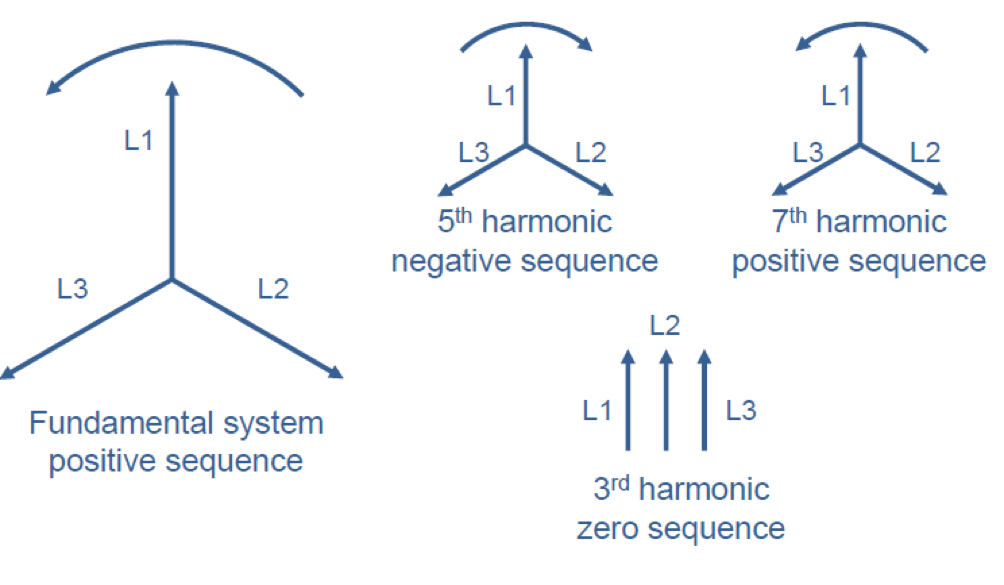# Basics of Harmonic Distortions (Part 2)

As the harmonic currents generated by the users’ equipment flow back towards the power source, they cause additional voltage distortion due to impedance associated with various power distribution equipment, such as transmission and distribution lines, transformers, cables, busbars, etc. Since inductive reactance is linearly proportional to frequency, especially high-order harmonic currents cause severe voltage distortion even with small current amplitudes:On the other hand, the amplitude of high frequency harmonic currents is usually relatively low. The harmonic components can be further classified as odd and even harmonics, non-integer harmonics and subharmonics. Most non-linear loads produce odd harmonics (i.e. 3rd, 5th, 7th, …). The production of even harmonics requires the existence of certain conditions, such as uneven current draw between the positive and negative half cycles. This can occur for instance with slightly imperfect firing angle control of thyristor rectifiers. Transformer magnetizing current and the starting current of arc furnaces also contain notable amounts of even harmonics.

Mostly harmonics of integer values are discussed, but also non-integer harmonics exist. Electric arc furnaces and arc welders are examples of loads that generate non-integer harmonics. Subharmonic currents have frequencies lower than the fundamental frequency, but they rarely exist in power systems. However, subharmonics may be generated if the power system is highly inductive or if there is a large power factor correcting capacitor bank connected.

In systems containing a lot of single-phase non-linear loads, triplen harmonics (i.e. harmonics that are multiples of three) can be a major problem. The phase sequences of different harmonics are presented in Figure 1.Figure 1: Phase sequence of harmonics

As can be seen from Figure 1, triplen harmonic currents are zero sequence components, i.e. instead of cancelling out each other in the star point they sum up in the neutral current. This is because the phase angle of the triplen harmonics is the same in all three phases.